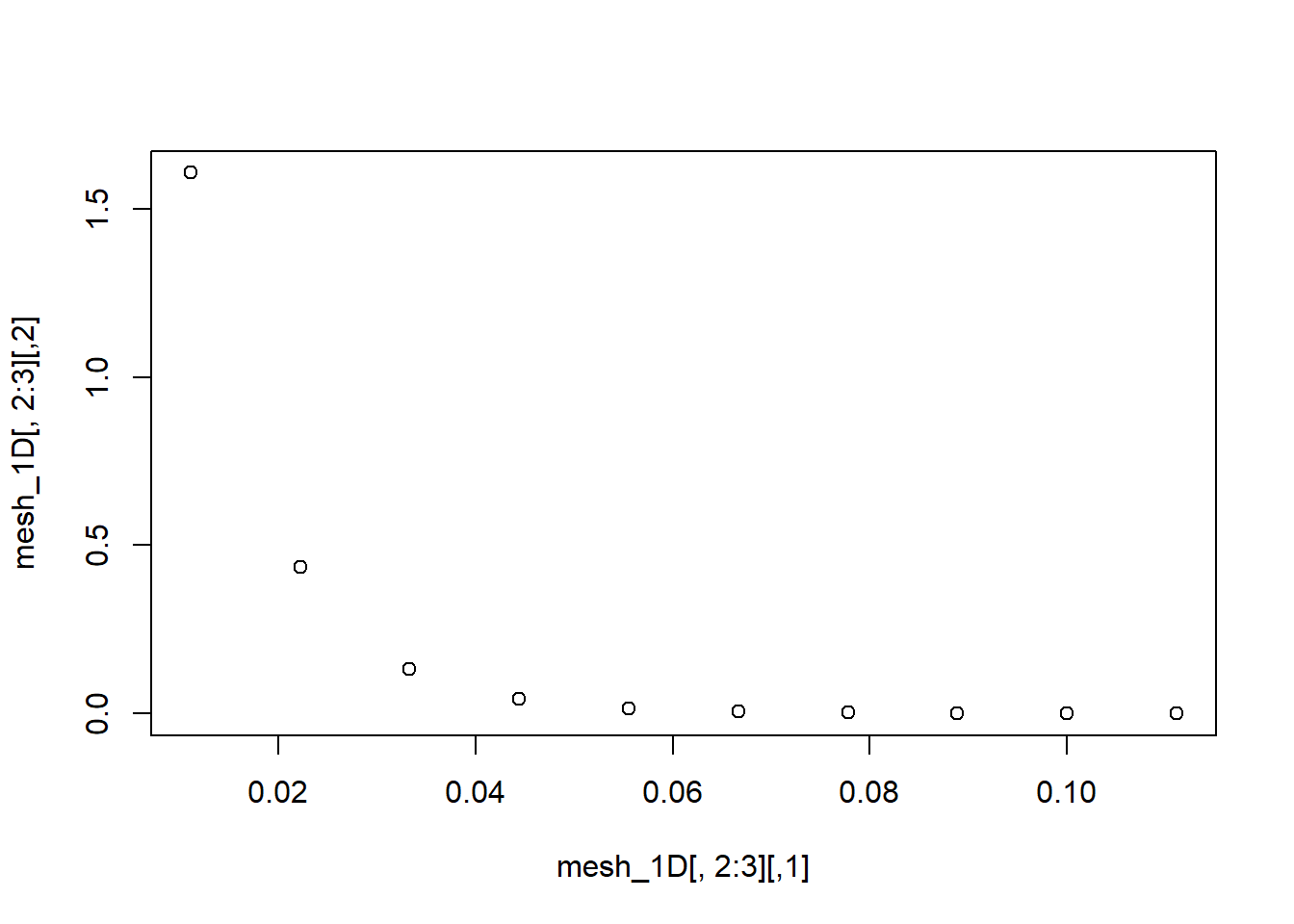# Mesh generation in CFD problem: An approach using Object Oriented Programming in R

## Background

I am currently working in understanding the water evaporation dynamics from soil. I read the paper of Kondo et al. The paper develop a model for calculating volumetric water content, specific humidity and temperature by mean of the solution of a system of PDE.

In order to solve the PDE, a solution approximation in finite differences is implemented. The algorith implementation involves a pre-processor module the which is conformed by fluid dynamics, boundary conditions and grid generation.

Provided boundary conditions, fluid dynamics equations and geometry, the grid can be generated. The objective was to develop a procedure for generating a mesh taking into account changes in geometry and boundary conditions

## Solution

I developed a class of type mesh, consisting of four attributes ( number of elements (ix), the compact matrix (mat), the number of nodes (nodes), and dimension order (dimension) ) and one method (print).

Several functions are required to set the class constructor: calculate the increment in every space dimension (x, y, or z) (xyz_dist); calculate the time increment to guarantee numerical stability (t_dis); set the value of boundary conditions (bound_cond), calculate the total number of element in mess (sum1toi)

Mesh generator requires an list as input parameter, consisting of dimension, xyz_dist, t_dist, boun_cond, and Courant sigma value. An instance of mesh class can be obtaining by passing the inparam list as argument, and result in matrix format can be obtained by mean of print.mesh method.

The mesh constructor and print method was based upon the class writing code read in the book The Art of R Programming by Norman Matloff

Mesh generation is tested for the 1D case. In the code shown below, the mesh for time intervals (column 1), 1D space (column 2), and boundary condition value (column 3). The mesh can be extended up to 3D by configuring the len_nod matrix in inparam list.

xyz_dist <- function(len_nod=matrix(data = c(c(rep(0.1,3)), c(rep(10,3))),nrow =2,ncol=3, byrow = TRUE) ){
len <- len_nod[1,]
innod <- len_nod[2,]
rtrn <- vector(mode="numeric", length = 3)
for (i in 1:length(rtrn)){
rtrn[i]<-len[i]/(innod[i]-1)
}
rtrn
}

t_dist <- function(xyz_d=vector(mode = "numeric",length = 3), sigma=0.5){
rtrn <- vector(mode = "numeric", length = 1)
for (i in 1:length(rtrn)){
rtrn[i] <- sigma * max(xyz_d)
}
rtrn
}

boun_cond <- function(theta=0.1, q=0.1, t=298.15){
return(1) #Dirichlet
}

sum1toi <- function(i,j) return(sum(i)*(j+2)) #i is mesh nodes vector, j is dimension

inparam <- list(di=1
,len_nod=matrix(data = c(c(rep(0.1,3)), c(rep(10,3))),nrow =2,ncol=3, byrow = TRUE)
,geom_dist = function(len_nod=matrix(data=0.0, nrow= 2,ncol=3) ){
len <- len_nod[1,]
innod <- len_nod[2,]
rtrn <- vector(mode="numeric", length = 3)
for (i in 1:length(rtrn)){
rtrn[i]<-len[i]/(innod[i]-1)
}
rtrn
}
,time_dist = function(xyz_d=vector(mode = "numeric",length = 3), sigma=0.5){
rtrn <- vector(mode = "numeric", length = 1)
for (i in 1:length(rtrn)){
rtrn[i] <- sigma * max(xyz_d)
}
rtrn
}
,bc = function(theta=0.1, q=0.1, t=298.15){
return(3.5e-05*(theta/0.4)**15) #Dirichlet
}
,sigma= 0.5)

mesh <- function(inparam){
# debug(inparam[])
rtrn <- list()
class(rtrn) <- "cdf_mesh"
spa_di <- inparam[]
lnods <- (inparam[])
n <- sum1toi(max(lnods),spa_di)
rtrn$mat <- vector(length= n) rtrn$ix <- (1:n)
rtrn$dimensions <- spa_di rtrn$nodes <- lnods[2,]
rtrn$n <- sum1toi(max(lnods[2,]),spa_di) fun_spa_dist <- inparam[] fun_t_dist <- inparam[] fun_bc <- inparam[] sigma <- inparam[] t_i <- 0 for (i in seq(1,n,spa_di+2)){ t_i <- t_i + fun_t_dist(fun_spa_dist(lnods),sigma) rtrn$mat[i]<- t_i
}
xyz_step <- 0
for (j in 1:spa_di){
for (i in seq(j+1,n,spa_di+2)){
xyz_step <- xyz_step +fun_spa_dist(lnods)[j]
rtrn$mat[i]<- xyz_step } for (i in seq(spa_di+2,n,spa_di+2)){ xyz_step <- xyz_step +fun_spa_dist(lnods)[j] theta <- 0.1/xyz_step rtrn$mat[i]<- fun_bc(theta=theta)
}
}
rtrn
}

print.mesh <- function(mesh){
m_n <- length(mesh$mat) geomdim <- mesh$dimension
n_col <- mesh$dimension+2 nodes <- max(mesh$nodes)
mat_vector <- mesh$mat n <- mesh$n
full_mesh <- matrix(nrow=nodes, ncol = n_col)
for (i in 1:(geomdim+2)) {
full_mesh[1:nodes,i] <- mat_vector[seq(i,m_n,n_col)]
}
return(full_mesh)
}

# Let's test the clas
mesh1 <- mesh(inparam)

# Print mesh
print.mesh(mesh1)
##              [,1]       [,2]         [,3]
##  [1,] 0.005555556 0.01111111 1.6066287663
##  [2,] 0.011111111 0.02222222 0.4355992520
##  [3,] 0.016666667 0.03333333 0.1311159516
##  [4,] 0.022222222 0.04444444 0.0431405106
##  [5,] 0.027777778 0.05555556 0.0153262862
##  [6,] 0.033333333 0.06666667 0.0058211136
##  [7,] 0.038888889 0.07777778 0.0023446175
##  [8,] 0.044444444 0.08888889 0.0009947598
##  [9,] 0.050000000 0.10000000 0.0004420810
## [10,] 0.055555556 0.11111111 0.0002048122
# Plot the boundary condition against x
mesh_1D <- print.mesh(mesh1)
plot(mesh_1D[,2:3])